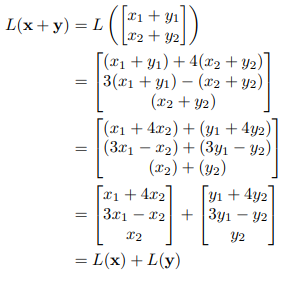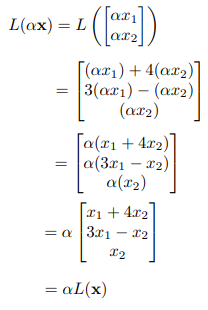# Linear Algebra

Linear algebra is one of the important branches of mathematics. Linear algebra is basically the study of vectors and linear functions. It is a key concept for almost all areas of mathematics. Linear algebra is considered a basic concept in the modern presentation of geometry. It is mostly used in Physics and Engineering as it helps to define the basic objects such as planes, lines and rotations of the object. It allows us to model many natural phenomena, and also it has a computing efficiency. In this article, you are going to learn about the basic introduction, its components, problems, linear equations and its applications.

## Introduction to Linear Algebra

Linear algebra is the study of linear combinations. It is the study of vector spaces, lines and planes, and some mappings that are required to perform the linear transformations.  It includes vectors, matrices and linear functions. It is the study of linear sets of equations and its transformation properties.

Linear Algebra Equations

The general linear equation is represented as

a1x1 + a2x2……….+anxn = b

Here,

a’s – represents the coefficients

x’s – represents the unknowns

b – represents the constant

There exists a system of linear algebraic equations, which is the set of equations. The system of equations can be solved using the matrices.

It obeys the linear function such as

(x1,……..xn) → a1x1 +……….+anxn

## Linear Algebra Topics

The most important topics covered in the linear algebra includes:

• Euclidean vector spaces
• Eigenvalues and eigenvectors
• Orthogonal matrices
• Linear transformations
• Projections
• Solving systems of equations with matrices
• Mathematical operations with matrices (i.e. addition, multiplication)
• Matrix inverses and determinants
• Positive-definite matrices
• Singular value decomposition
• Linear dependence and independence

Here, the three main concepts which are the prerequisite to linear algebra are explained in detail. They are:

• Vector spaces
• Linear Functions
• Matrix

All these three concepts are interrelated such that a system of linear equations can be represented using these concepts mathematically. In general terms, vectors are elements that we can add, and linear functions are the functions of vectors that include the addition of vectors

## Vector Spaces

As we know that linear algebra deals with the study of vector spaces and the linear transformations between them. By the definition of vector, it is a physical quantity that has both magnitude and direction. A vector space is defined as the collection of objects called vectors, which may be added together and multiplied (i.e. scaled) by numbers, called scalars. Generally, real numbers are taken as scalars, but there exist vector spaces with scalar multiplication by non-real numbers, i.e. complex numbers, or naturally any field.

The operations such as vector addition and scalar multiplication must satisfy specific requirements, called vector axioms. Generally, the terms real vector space and complex vector space are used to define that the scalars are real or complex numbers, respectively.

Suppose V be any vector space with elements a, b, c and scalars m, n over a field F, then the vector axioms are given by:

• Commutative of addition: a + b = b + a
• Associativity of addition: a + (b + c) = (a + b) + c
• Additive identity: a + 0 = 0 + a = a, where 0 is an element in V called zero vector.
• Additive inverse: a + (-a) + (-a) + a = 0, a, -a belongs to V.

These four axioms define that the vector space V is an abelian group under addition.

Other axioms include distributivity of scalar multiplication with respect to vector addition and field addition, identity element of scalar multiplication and so on.

For example, m(a) = ma; n(a + b) = na + nb

An element of a specific vector space may have different characteristics. For example, the elements can be a sequence, a function, a polynomial or a matrix. Linear algebra is affected by those properties of such things that are common or familiar to all vector spaces.

A linear map can be written for two given vector spaces namely V and W over a field F. This is sometimes referred to as linear transformation or mapping of vector spaces. Thus, it is given by:

T: V → W

This allows us to write the addition of scalar multiplication of elements such as:

T(a + b) = T(a) + T(b)

T(ma) = mT(a)

## Linear Function

A linear function is an algebraic equation in which each term is either a constant or the product of a constant and a single independent variable of power 1. In linear algebra, vectors are taken while forming linear functions. Some of the examples of the kinds of vectors that can be rephrased in terms of the function of vectors.

Mathematically, linear function is defined as:

A function L : Rn → Rm is linear if

(i) L(x + y) = L(x) + L(y)

(ii) L(αx) = αL(x)

for all x, y ∈ Rn, α ∈ R

Example:

$$\begin{array}{l}\text{Show that the function }L : R^2 → R^3 \text{ given by } L(x)=\begin{bmatrix} x_1+4x_2\\ 3x_1-x_2\\ x_2 \end{bmatrix} \text{ is linear. }\end{array}$$

Solution:

For any x, y ∈ R2, we haveFor any x ∈ R2 and α ∈ R, we haveTherefore, L is a linear function.

## Linear Algebra Matrix

Matrices are linear functions of a certain kind. Matrix is the result of organizing information related to certain linear functions. Matrix almost appears in linear algebra because it is the central information of linear algebra.

Mathematically, this relation can be defined as follows.

A is an m × n matrix, then we get a linear function L : Rn → Rm by defining

L(x) = Ax

or

Ax = B

Go through the example given below to understand this mapping in detail.

Question:

A room contains x bags and y boxes of fruits and each bag contain 2 apples and 4 bananas and each box contains 6 apples and 8 bananas. There are a total of 20 apples and 28 bananas in the room. Find the value of x and y.

Solution :

Write the simultaneous equation for the given information that the above condition becomes true.

2x + 6y = 20

4x + 8y = 28

Here the example given above shows the system of linear equations.

Now, write the above equation as equality between 2- vectors and using the rules, we get

$$\begin{array}{l}\begin{pmatrix} 2x+6y\\ 4x+8y \end{pmatrix}= \begin{pmatrix} 20\\ 28 \end{pmatrix}\end{array}$$
$$\begin{array}{l}x\begin{pmatrix} 2\\ 4 \end{pmatrix}+y\begin{pmatrix} 6\\ 8 \end{pmatrix}=\begin{pmatrix} 20\\ 28 \end{pmatrix}\end{array}$$

We denote the functions as an array of numbers is called a matrix.

$$\begin{array}{l}\text{Therefore, the function }\begin{pmatrix} 2 &6 \\ 4& 8 \end{pmatrix} \text{ is defined by}\end{array}$$
$$\begin{array}{l}\begin{pmatrix} 2 &6 \\ 4& 8 \end{pmatrix}\begin{pmatrix} x\\ y \end{pmatrix}=x\begin{pmatrix} 2\\ 4 \end{pmatrix}+y\begin{pmatrix} 6\\ 8 \end{pmatrix}\end{array}$$

## Numerical Linear Algebra

Numerical linear algebra is also known as the applied linear algebra. Applied linear algebra deals with the study of how matrix operations can be used to create computer algorithms, which helps to solve the problems in continuous mathematics with efficiency and accuracy.  In numerical linear algebra, many matrix decomposition methods are used to find the solutions for common linear algebraic problems like least-square optimization, locating Eigenvalues, and solving systems of linear equations. Some of the matrix decomposition methods in numerical linear algebra include Eigen decomposition, Single value decomposition, QR factorization and so on.

## Linear Algebra Applications

Here, some of the linear algebra applications are given as:

• Ranking in Search Engines – One of the most important applications of linear algebra is in the creation of Google. The most complicated ranking algorithm is created with the help of linear algebra.
• Signal Analysis – It is massively used in encoding, analyzing and manipulating the signals that can be either audio, video or images etc.
• Linear Programming – Optimization is an important application of linear algebra which is widely used in the field of linear programming.
• Error-Correcting Codes – It is used in coding theory. If encoded data is tampered with a little bit and with the help of linear algebra it should be recovered. One such important error-correcting code is called hamming code
• Prediction – Predictions of some objects should be found using linear models which are developed using linear algebra.
• Facial Recognition- An automated facial recognition technology that uses linear algebraic expression is called principal component analysis.
• Graphics- An important part of graphics is projecting a 3-dimensional scene on a 2-dimensional screen which is handled only by linear maps which are explained by linear algebra.

### Linear Algebra Problems

Linear algebra problems include matrices, spaces, vectors, determinants, and a system of linear equation concepts. Now, let us discuss how to solve linear algebra problems.

Example 1:

Find the value of x, y and z for the given system of linear equations.

2x + y – z = 8

-3x – y + 2z = -11

-2x + y + 2z = -3

Solution:

Given,

2x + y – z = 8

-3x – y + 2z = -11

-2x + y + 2z = -3

The matrix is of the form,

AX = B,

Here ,

$$\begin{array}{l}A= \begin{bmatrix} 2 & 1& -1\\ -3 & -1& 2\\ -2 & 1 &2 \end{bmatrix}\end{array}$$
$$\begin{array}{l}x=\begin{bmatrix} x\\ y\\ z \end{bmatrix}\end{array}$$
$$\begin{array}{l}B=\begin{bmatrix} 8\\ -11\\ -3 \end{bmatrix}\end{array}$$

After performing elementary row operation and augmented matrix, it is reduced to the form

$$\begin{array}{l}A \begin{bmatrix} 2 & 1 & -1 & 8\\ -3 & -1 & 2 & -11\\ -2 & 1 & 2 & -3 \end{bmatrix}\end{array}$$

Now the reduced echelon form of the above matrix is,

$$\begin{array}{l}A \begin{bmatrix} 1& 0 & 0& 2\\ 0 & 1 & 0 & 3\\ 0 & 0 & 1 & -1 \end{bmatrix}\end{array}$$

Therefore, the unique solution for this is,

x = 2

y = 3

z = -1

Example 2:

$$\begin{array}{l}\text{Find the value of x, given that }\begin{vmatrix} 3 & x \\ x & 1 \end{vmatrix} =\begin{vmatrix} 3 & 2\\ 4 & 1 \end{vmatrix}\end{array}$$

Solution:

Given that:

$$\begin{array}{l}\begin{vmatrix} 3 & x \\ x & 1 \end{vmatrix} =\begin{vmatrix} 3 & 2\\ 4 & 1 \end{vmatrix}\end{array}$$

Thus, the above determinant can be equated as:

3 – x2 = 3 – 8

3 – x2 = -5

-x2 = -5-3

-x2 = -8,

Now, multiply both sides by -1, we get

x2 = 8

x = ± 22

Therefore, the value of x is ± 22.

Also, learn: Solving Linear Equations

### Practice Problems on Linear Algebra

1. Solve the following pair of linear equations using the substitution method: 7x-15y=2, x+2y=3.
2. Solve the equations 2x+3y=11, and 2x-4y=-24. Also, determine the value of “m” for which y=mx+3.
3. Solve the following equations using the substitution method and elimination methods: 3x + 4y = 10 and 2x – 2y = 2.

For more related articles on the system of linear equations, register with BYJU’S – The Learning app and watch interactive videos.

## Frequently Asked Questions on Linear Algebra

Q1

### What is meant by linear algebra?

Linear algebra is the study of lines, planes, vector spaces, mappings, which are required for the linear transformation.

Q2

### Mention the applications of linear algebra?

Some of the real-life applications of linear algebra include facial recognition, testing of coding in software engineering, ranks in the search engine, graphics and so on.

Q3

### What is called a linear function?

A linear function is a kind of algebraic equation, in which each term is a constant or a product of constant and a single independent variable of power 1.

Q4

### What is meant by a vector?

A vector is a quantity that has two independent properties called magnitude and direction.

Q5

### What is meant by vector space?

A vector space consists of a set of objects called vectors, which can be added together and multiplied by the numbers called scalars.